# 5TH ANNIVERSARY AUCTION

### Auction Details

Choose Your Layout >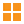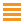242 - Shelf One
CDC Auctions
Shelf One
Bidding Has Concluded

Sold to f***r for (17.50 + 2.28) x 1 = 19.78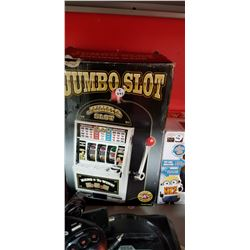243 - Shelf One
CDC Auctions
Shelf One
Bidding Has Concluded

Sold to f***r for (15.00 + 1.95) x 1 = 16.95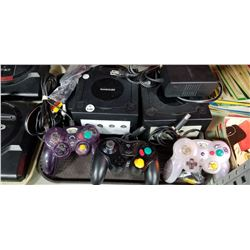244 - Shelf One
CDC Auctions
Shelf One
Bidding Has Concluded

Sold to f***r for (40.00 + 5.20) x 1 = 45.20245 - Shelf One
CDC Auctions
Shelf One
Bidding Has Concluded

Sold to j*****R for (17.50 + 2.28) x 1 = 19.78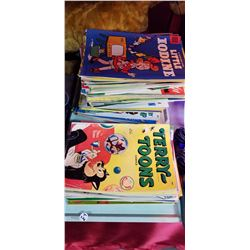246 - Shelf One
CDC Auctions
Shelf One
Bidding Has Concluded

Sold to f***r for (75.00 + 9.75) x 1 = 84.75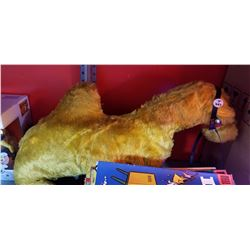247 - Shelf One
CDC Auctions
Shelf One
Bidding Has Concluded

Sold to f***r for (12.50 + 1.63) x 1 = 14.13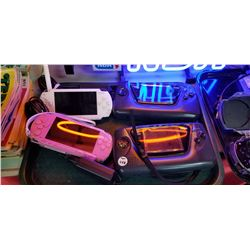248 - Shelf One
CDC Auctions
Shelf One
Bidding Has Concluded

Sold to f***r for (37.50 + 4.88) x 1 = 42.38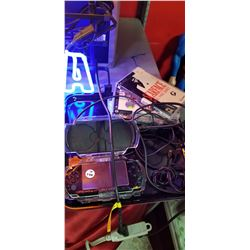249 - Shelf One
CDC Auctions
Shelf One
Bidding Has Concluded

Sold to f***r for (60.00 + 7.80) x 1 = 67.80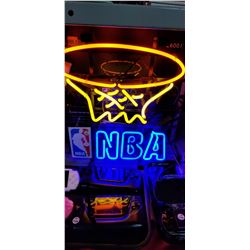250 - Shelf One
CDC Auctions
Shelf One
Bidding Has Concluded

Sold to f***r for (95.00 + 12.35) x 1 = 107.35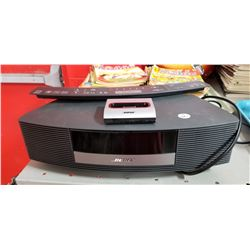251 - Shelf Two
CDC Auctions
Shelf Two
Bidding Has Concluded

Sold to f***r for (110.00 + 14.30) x 1 = 124.30252 - Shelf One
CDC Auctions
Shelf One
Bidding Has Concluded

Sold to f***r for (90.00 + 11.70) x 1 = 101.70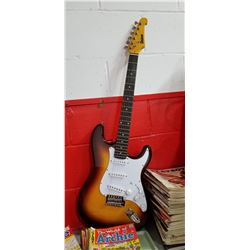253 - Shelf One
CDC Auctions
Shelf One
Bidding Has Concluded

Sold to f***r for (85.00 + 11.05) x 1 = 96.05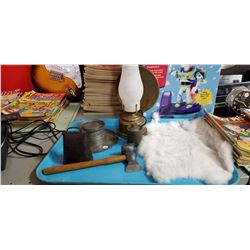254 - Shelf One
CDC Auctions
Shelf One
Bidding Has Concluded

Sold to f***r for (20.00 + 2.60) x 1 = 22.60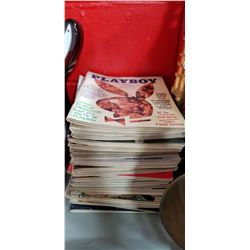255 - Shelf One
CDC Auctions
Shelf One
Bidding Has Concluded

Sold to s********F for (15.00 + 1.95) x 1 = 16.95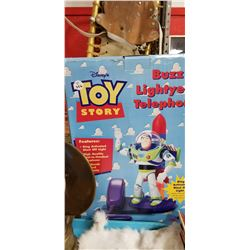256 - Shelf One
CDC Auctions
Shelf One
Bidding Has Concluded

Sold to f***r for (22.50 + 2.93) x 1 = 25.43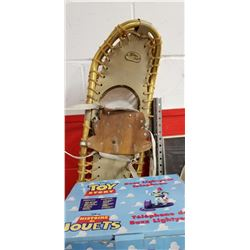257 - Shelf One
CDC Auctions
Shelf One
Bidding Has Concluded

Sold to f***r for (30.00 + 3.90) x 1 = 33.90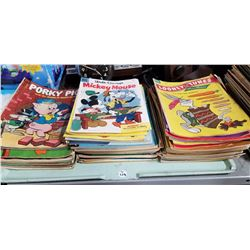258 - Shelf One
CDC Auctions
Shelf One
Bidding Has Concluded

Sold to f***r for (50.00 + 6.50) x 1 = 56.50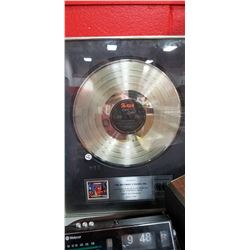260 - Shelf One
CDC Auctions
Shelf One
Bidding Has Concluded

Sold to f***r for (45.00 + 5.85) x 1 = 50.85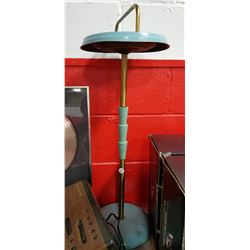261 - Shelf One
CDC Auctions
Shelf One
Bidding Has Concluded

Sold to f***r for (12.50 + 1.63) x 1 = 14.13